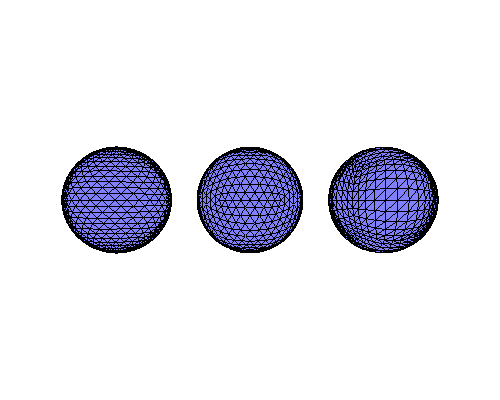# Vispy example¶

Note that this example depends on the Vispy library, and that you need a bleeding edge version of Vispy to run this.

An example showing how jupyter_rfb is used in Vispy. Note that Vispy implements a subclass of RemoteFrameBuffer for this to work.

:

from IPython.display import display
from vispy import scene
from vispy.visuals.transforms import STTransform

canvas = scene.SceneCanvas(keys='interactive', bgcolor='white',
size=(500, 400), show=True, resizable=True)

view.camera = 'arcball'

edge_color='black')

edge_color='black')

sphere3 = scene.visuals.Sphere(radius=1, rows=10, cols=10, depth=10,
method='cube', parent=view.scene,
edge_color='black')

sphere1.transform = STTransform(translate=[-2.5, 0, 0])
sphere3.transform = STTransform(translate=[2.5, 0, 0])

view.camera.set_range(x=[-3, 3])
canvas

:snapshot
[ ]: